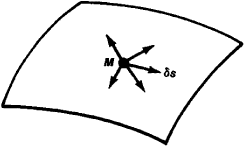# Virtual Displacements

The following article is from The Great Soviet Encyclopedia (1979). It might be outdated or ideologically biased.

## Virtual Displacements

elementary (infinitely small) displacements that a point in a mechanical system can undergo from the position occupied by it at a given moment in time without violating the constraints imposed on the system. Virtual displacement is a purely geometric concept and does not depend on actual forces. It is determined only by the type of constraints imposed on the system and is introduced as a property of these constraints, showing what displacements are possible in the system under the imposed constraints. For example, if a surface is the constraint for a point and the point is in position M on the surface at a given moment (see Figure 1), then the virtual displacementsFigure 1

of the point at that moment will be elementary segments (vectors) of length δs along the tangent to the surface at point M. Displacement in any other direction would not be virtual displacement, since it would violate the constraint (the point would no longer be on the surface). The concept of virtual displacement applies both to a resting and a moving point. If a constraint does not change with time, then the true elementary displacement ds of a moving point from position M coincides with a virtual displacement.

The concept of virtual displacement is used to determine the conditions of equilibrium and equations of motion of a mechanical system and also to find the number of degrees of freedom in a system.

S. M. TARG

The Great Soviet Encyclopedia, 3rd Edition (1970-1979). © 2010 The Gale Group, Inc. All rights reserved.
References in periodicals archive ?
Another reason is that there exist some errors and virtual displacements in test inevitably, which leads to the test values larger.
All the terms in the weak form have now been written in terms of unknown coordinates and virtual displacements. Collecting the terms gives
Since the virtual displacements are arbitrary, the [[??].sub.B] value is in accordance with
The above relation should hold for arbitrary virtual displacements [delta]w, [delta]v, and [delta][phi] which reaches the dynamic equilibrium equation set of FGM rings, by combining the virtual form of [[epsilon].sub.[theta][theta]0], [[epsilon].sub.[theta][theta]1], and [y.sub.r[theta]0] with (11) and then utilizing the procedure of part integration.
On the basis of the principle of virtual displacements, then the governing equations are obtained 
The principle of virtual displacements formulation for the dynamics equilibrium equation at t = t + [DELTA]t for the fiber can be written as
The principle of virtual work states that the variation of work W induced by forces [F.sub.i] and virtual displacements [du.sub.i] in an equilibrium state equals zero:
After a review of Newtonian dynamics, the basic concepts of analytical dynamics--classification of constraints, classification of forces, virtual displacements, virtual work, and variational principles--are introduced and developed.
Since Galerkin's method uses the same approximation for both field variable w and weighting function N, by applying Green's theorem (4) to the above area integrals twice and taking the conformity of virtual displacements and loads across element boundaries into consideration, one can change Galerkin's integral equation (3) to its equivalent weak form:
Elements nodal vectors [u.sub.n.sup.e], [delta][u.sub.n.sup.e], and [X.sub.n.sup.e] represent respectively the real displacements, the compatible virtual displacements, and positions.
Calculation of the first generalized force [mathematical expression not reproducible] requires the imposition of the first independent virtual displacement [delta]([x.sub.1]) [not equal to] 0 to the mechanical system in Figure 6 with simultaneous "freezing" or "sticking down" of cart B to the upper rails and "freezing" or "sticking to the left" of cable AE to the vertical axis z factually assuming zero-values of another two virtual displacements [delta]([x.sub.2]) = 0 and [delta]([alpha]) = 0:
The stiffness and mass matrices of the elements were obtained via the principle of virtual displacements as follows:

Site: Follow: Share:
Open / Close